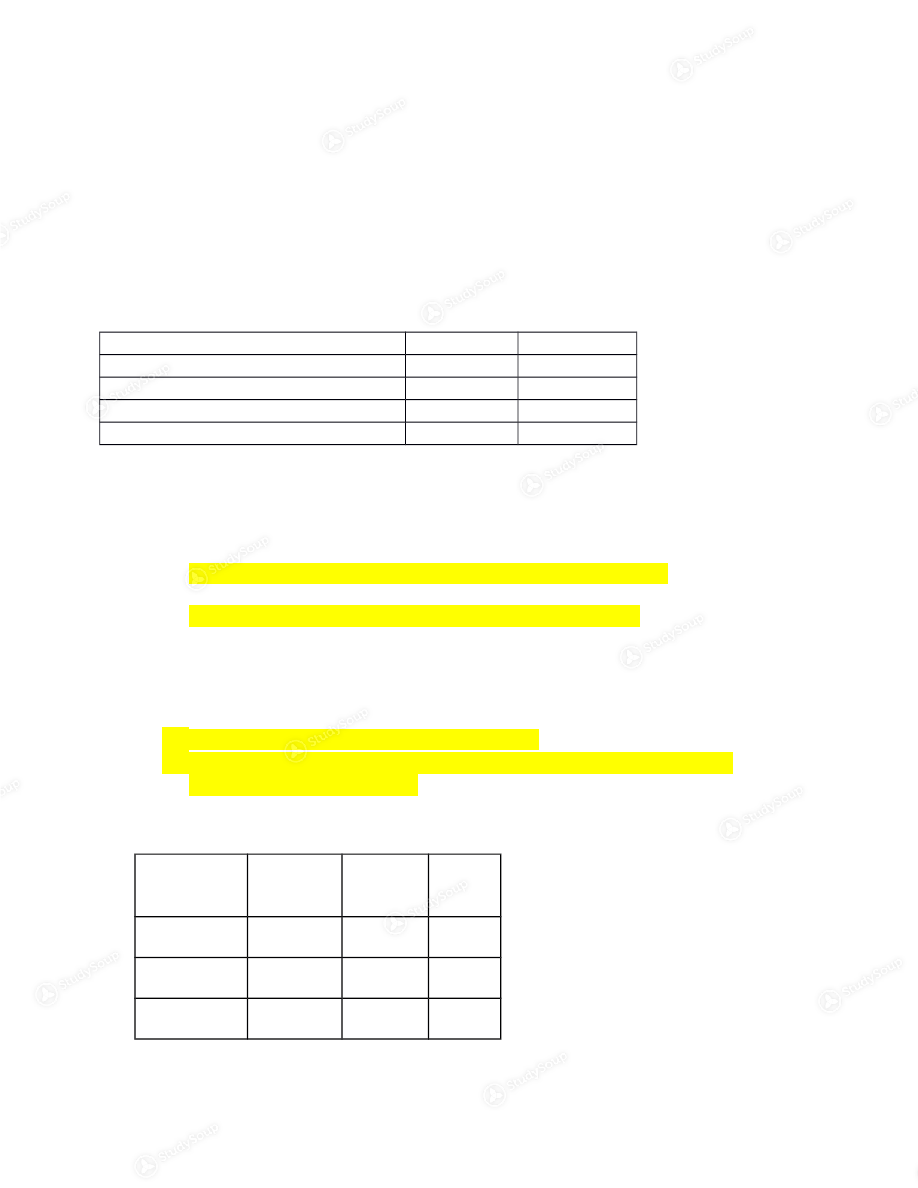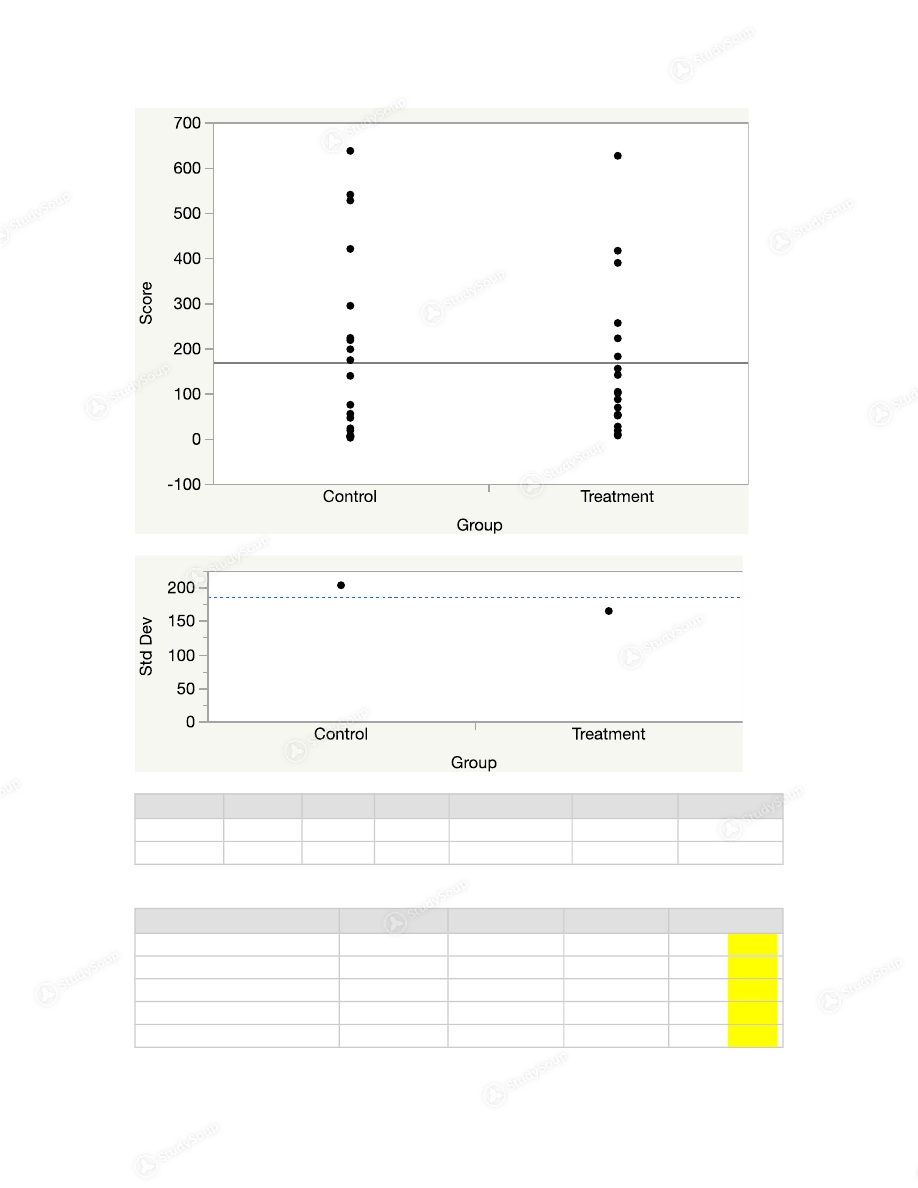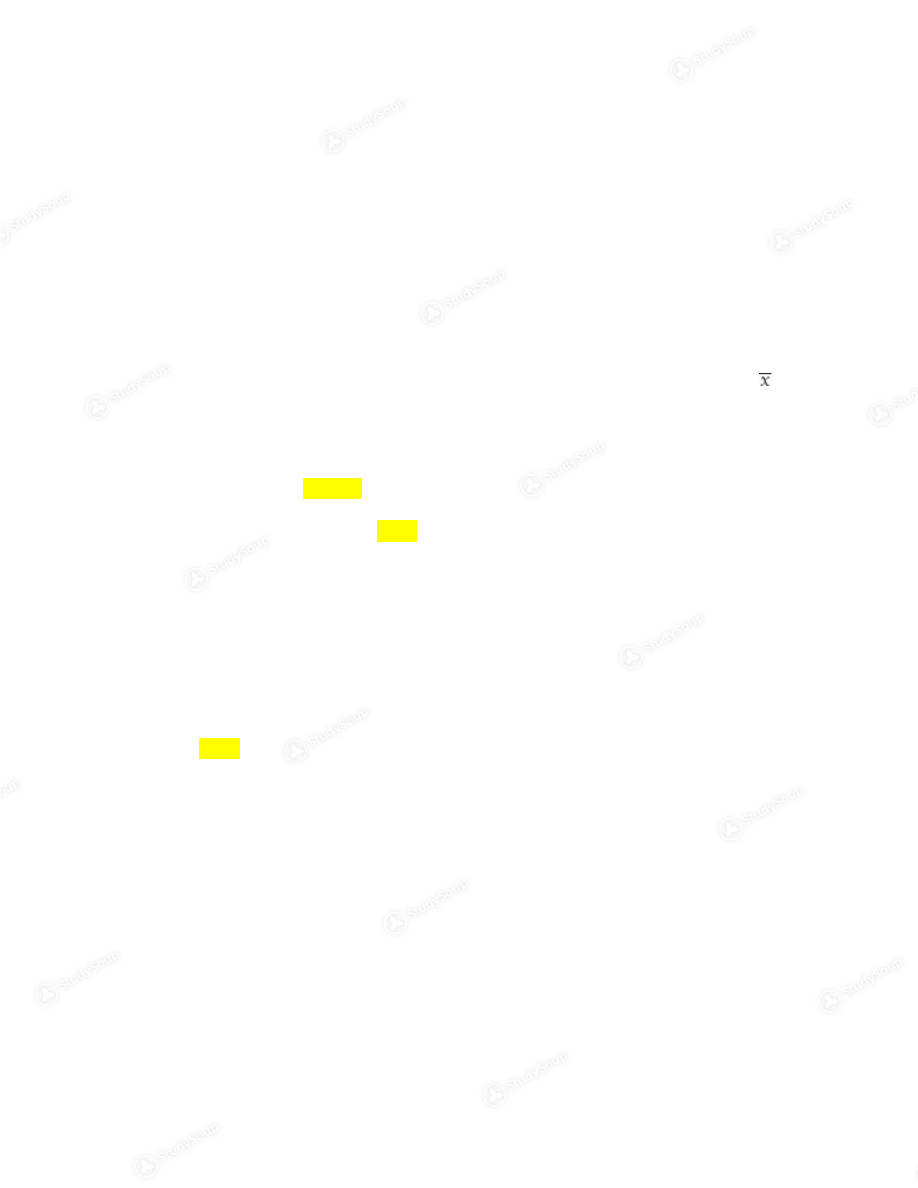Limited time offer 20% OFF StudySoup Subscription details

# Purdue - STAT 50300 - Stat Homework - Class Notes

### Created by: kmr18 Elite Notetaker

> > > > Purdue - STAT 50300 - Stat Homework - Class Notes

Purdue - STAT 50300 - Stat Homework - Class Notes

##### Description: This is the homework assignments for stat 50300
This preview shows pages 1 - 9 of a 75 page document. to view the rest of the contentStatistics 503, Practical Skill Test  Time:   75 minutes Name:  Section:   05     02        online  In this exam, you should answer the questions using JMP,  attach relevant JMP output,  then highlight your answer. The format should be similar to the PQs in the homework.  Specifically for all hypothesis testing questions.  i) Define the Hypothesis with correct symbols.  ii) Perform an appropriate assumption checking on data. ii)         Perform the test, show the relevant JMP output, and highlight your answer.
iii)
State the conclusion in the context of the problem.  This is an open note, open book exam. But it is an independent exam meaning you must
complete on your own and cannot discuss, share or collaborate with anyone who is
or isn’t taking this exam.  You will have  two chances to submit your exam, but only the final submission will be graded. Submission is done online. Exams submitted after 9:15pm will not be graded. Please sign below to indicate your agreement with the following honour code.
Honour code:  I have read through the above instructions. I promise not to cheat on
this exam.  I will neither give nor receive any unauthorized assistance.  I promise
not to share information about this exam with anyone who may be taking it at a
different time.  I have not been told anything about the exam by someone who has
Initial:__KR__          Date: __11/30/2017__ Proble m Total Actual  1 10   2 10   Total  201. Researchers in Germany examined the link between pigmentation (freckles) and reaction   to   sun   exposure.   After   examining   the   reaction   (response)   to   midday summer   sun   exposure   for   thousands   of   Caucasian   children,   they   found   the
following distribution in their sample.
a) Describe the variables and samples in the experiment.  Ho: the reaction type to sun is not associated with freckle situation Ha: the reaction type to sun is associated with freckle situation Assumption checking:  Each observation is independent of all the others No more than 20% of the expected counts are less than 5 and all individual
expected counts are 1 or greater
The expected frequencies are Freckles No  Freckles Total I 114.105 37.895 152 II 711.6572 236.3428 948 III 1012.685 336.315 1349 1 Reaction type to sun No freckles  Freckles (I)Always reddens, never tans 79 73 (II)Always reddens, slightly tans 581 367 (III)Sometimes reddens, always tans 1025 324 (IV) Never reddens, always tans 1022 135IV 868.552 288.448 1157 Total 2706.9992 899.0008 3606 b) Perform a hypothesis test to find out whether reaction type to sun is associated  with freckle situation? (at  α=0.05 )    The chisquare contributions are Oi Ei (Oi­ Ei)^2 /Ei 79 114.105 10.8002 73 37.895 32.5204 581 711.6572 23.9881 367 236.3428 72.2311 1025 1012.685 0.1498 324 336.315 0.4509 1022 868.552 27.1098 135 288.448 81.631 Total: 248.8813 Pigmentation By Reaction Type Count
Expected
Cell Chi^2 I II III IV Total Freckles 79 114.105 10.8004 581 711.657 23.9881 1025 1012.69 0.1498 1022 868.552 27.1098 2707 No Freckles 73 37.8946 32.5214 367 236.343 72.2312 324 336.315 0.4509 135 288.448 81.6309 899 Total 152 948 1349 1157 3606 2Degrees of freedom: 3 Test Statistic, X^2: 248.8825
Critical X^2: 7.814736
P­Value: 0.0001 Test ChiSquare Prob>ChiSq Likelihood Ratio 253.091 <.0001* Pearson 248.882 <.0001* Here P­value 0.0001 < alpha 0.05, So we reject Ho. Thus, we conclude that the
reaction type to sun is associated with freckle situation
c) Perform a hypothesis test to test whether the proportion of type IV reaction in the freckles condition (i.e., children with freckles) is significantly less than 18% (in
other words, the proportion of type I, II, or III reaction in the children with
freckles is significantly more than 82%).   (at  α=0.05 ) Ho: P = 0.18 and Ha: P < 0.18 Assumption checking: Random sampling from a define population Normally distributed Let the LOS be alpha 5%, Rearrange the data for sample proportion, No
Freckles
freckles Total I, II,
III
1685 764 2449 IV 1022 135 1157 Total: 2707 899 Sample proportion p = 135/899 = 0.1502 3Test Statistic: Z=p­P/sqrt(PQ/n)
=0.1502 – 0.18/sqrt(.18*.82)/899
= ­2.3283   z Test Test Statistic 8484.72 Prob > |z| <.0001* Prob > z <.0001* Prob < z 1.0000 Critical z: ­1.6449
P­Value: 0.0001
Here P­value 0.0001 < alpha 0.05, we reject Ho. Thus, we conclude that the freckles
condition (i.e., children with freckles) is significantly less than 18%
2. Snoring is an extremely common and burdensome condition occurring when  vibrating soft tissue obstructs the upper airway during sleep. Researchers
investigated the eﬀectiveness of throat exercises designed to strength that soft
medical care for a primary complaint of snoring. At random, the subjects were di­
vided into two treatment groups lasting for three months. The control group was
asked to perform deep breathing exercises daily and to sleep with a nasal dilator
strip. The therapy group was instructed to perform a series of throat exercises
three times a day. After these three months, subjects were recorded during sleep
to assess their snoring frequency (in number of snores per hour). Here are the
ﬁndings: control 2 4 6 6 6 18 23 46 55 75 139 174 198 218 223 294 420 527 540 637 treatmen t 7 10 18 27 51 52 53 69 87 101 104 141 155 182 222 256 389 416 626   Does the study provide signiﬁcant evidence that throat exercises are better than nasal
strips and breathing exercises in addressing snoring in this population? (at
α=0.05 H 0 T  =  C H a T  >  C Perform an independent two sample T test.  Assumption testing: test Normality for the control  4Level Number Mean Std Dev Std Err Mean Lower 95% Upper 95% Control 20 180.550 203.103 45.415 85.495 275.61 Treatment 19 156.105 164.614 37.765 76.764 235.45 Test F Ratio DFNum DFDen p­Value O'Brien[.5] 0.6149 1 37 0.4379 Brown­Forsythe 1.0266 1 37 0.3175 Levene 1.2095 1 37 0.2785 Bartlett 0.7859 1 . 0.3753 F Test 2­sided 1.5223 19 18 0.3777 5Welch Anova testing Means Equal, allowing Std Devs Not Equal F Ratio DFNum DFDen Prob > F 0.1713 1 36.127 0.6814 t Test 0.4139 The pvalues are greater than 0.05, hence we use the pooled method.  The Welch’s test, pvalue=0.6814, conclude that the treatment has no significant effect.  Or perform a pooled test. As the result shows below, pvalue=0.6814, conclude that the  treatment has no significant effect.  T test Treatment­Control
Assuming equal variances
Difference  ­24.44 t Ratio  ­0.41386 Std Err Dif 59.07 DF 36.12719 Upper CL Dif 95.33 Prob > |t| 0.6814 Lower CL Dif  ­144.22 Prob > t 0.6593 Confidence 0.95 Prob < t 0.3407 6Homework 8 (50pts) Due 10/27 1. (This is also the  Sweetening cola example in topic 8): calculating power. The  cola maker wants to test at the 5% significance level the following hypotheses: H 0 μ = 0 versus H a μ > 0 Ten taste scores were used for the significance test. The distribution of taste
scores is assumed to be roughly Normal with standard deviation σ = 1. We want
to calculate the power of this test when the true mean sweetness loss is μ = 0.8.
(a) What values of the z statistic would lead us to reject H 0 ? To what values of  do they correspond? Use the inverse Normal calculations to figure this out. z=xbar­mu/(sigma/(sqrtn)) reject Ho when z=1.645 xbar>1.645(1/sqrt10)+.8=1.320 (b) When the true mean sweetness loss is μ = 0.8, how often would we reject H 0 That is, what is the probability of obtaining sample averages like the ones defined
in (a) when μ = 0.8? Use the z table to calculate this probability. This probability
is the power of your test against the alternative μ = 0.8, the probability of rejecting
H
0  when the alternative μ = 0.8 is true. Pr(z>1.320 given mu = .8)
1­.9495
=.0505 2. In order to test a hypothesis Ho:  μ=5   Ha:  μ <5 , a sample of subjects were randomly selected. Suppose the population is Normal  with  σ =0.92  mg/l.   a) Compute the power of the test ( α=0.05 ¿ if the sample size is 45 and the  true mean is 4.75.   Ho:  μ=5   Ha:  μ <5 z<­1.645 xbar<4.774
power: P(xbar<4.774 given mu=4.75)
P(z<.17).5675 b) Compute the power of the test ( α=0.05 ) if the sample size is 60 and the  true mean is 4.75.  Ho:  μ=5   Ha:  μ <5 z<­1.645 xbar<4.805
power: P(xbar<4.805 given mu=4.75)
P(z<.46)
.6772
c) Compute the power of the test if  α=0.1  if the sample size is 45 and the  true mean is 4.75.  Ho:  μ=5   Ha:  μ <5 z<­1.282
xbar<4.824
power: P(xbar<4.824 given mu=4.75)
P(z<.54)
.7054 3. In a study of the nutritional requirements of cattle, researchers measured the weight
gains of cows during a 78­day period. For two breeds of cows, Hereford(HH) and Brown
Swiss/Hereford(SH), the results are summarized in the following table. (Note, the precise
df is 71.9).
HH SH n 33 51   18.3 13.9 s 17.8 19.1 a) Perform a two sample t test with the unpooled SE to compare the means. Use  =0.1.  α Make sure you   Define Ho/Ha Find test statistics Estimate p­value State conclusion  List the conditions for the test (for example, should the two samples be normally
distributed? Should HH data and SH data be independent?)
Ho: u1=u2 Ha: u1=/= u2 df= 71.9
level of significance= .1
Y

This is the end of the preview. Please to view the rest of the contentJoin more than 18,000+ college students at Purdue University who use StudySoup to get ahead
75 Pages 199 Views 159 Unlocks
• Notes, Study Guides, Flashcards + More!Join more than 18,000+ college students at Purdue University who use StudySoup to get ahead
##### Description: This is the homework assignments for stat 50300
75 Pages 199 Views 159 Unlocks
• Notes, Study Guides, Flashcards + More!
×

I don't want to reset my password

Need help? Contact support

Need an Account? Is not associated with an account
We're here to help